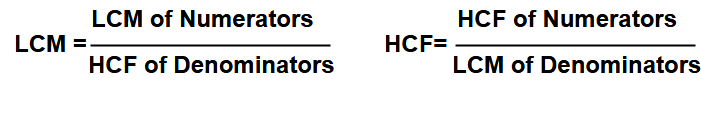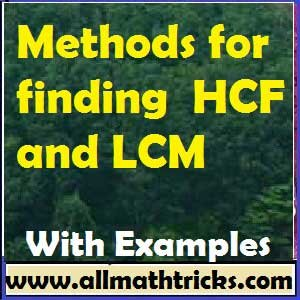# Typical problems on HCF and LCM | GCD and LCM Problems & Solutions

## Practice problems on HCF and LCM for all competitive exams

Common Divisor : A number which exactly divides all the given numbers is a common divisor of those numbers.

For example ” 3″ is common divisor of 9, 12, 15, 18.

Greatest Common Divisor(GCD) or Highest Common factor ( HCF )  : The greatest number that exactly divides all the given number is the GCD or HCF of those numbers

Common Multiple : A number which is exactly divisible by all the given numbers is a common multiple of those numbers.

For example ” 15 ” is a common multiple of 3 and 5.

Least Common Multiple (LCM) : The lowest number which is exactly divisible by all the given numbers is the LCM of those numbers.

The concepts and methods to find Lowest Common Multiple (LCM) and Highest Common Factor (HCF ) explained in the below link

Concepts of Highest Common Factor (Greatest common divisor –  GCD) and Lowest Common Multiple | Factorization and Division Methods for finding HCF and LCM.

In this page explained different types  of typical problems with solutions on HCF ( GCD ) & LCM

Some Important tips in HCF and LCM.

a) Find the least number which when divided by n1 , n2 & n3 leaves the remainders x ,y , and z respectively. Here answer is [ LCM of (n1, n2, n3) – k ] where k =  (n1 -x) = (n2 -y) = (n3 -z).

b) Find the least number which when divided by n1 , n2 & n3 leaves the same remainder ” k” . Here answer is [ LCM of (n1, n2, n3) + k ]

c) Find the greatest number which exactly divide  n1 , n2 & n3 leaves the same remainder ” k” . Here answer is [ HCF of (n1-k, n2-k, n3-k).

d) Find the greatest number which exactly divide  n1 , n2 & n3 leaves the same remainder  x, y and z respectively . Here answer is [ HCF of (n1-x, n2-y, n3-z).

e) GCD (m, n ) x LCM(m, n) = m x n ( i.e The product of HCF and the LCM equals the product of the numbers.

f) HCF of fractions and LCM of fractions.Example – 1 : What is the side of largest possible square slab which can be paved on the floor of a room 2m 73cm long and 3m 25cm broad?

Sol : Here possible largest square slab means it equal to greatest common divisor of both sides i.e 2m 73cm and 3m 25cm.

i.e 2m 73cm = 273cm & 3m 25cm = 325cm. Now find the GCD for 273 and 325.

273 = 131 x 71 x 31

325 = 131 x 52

GCD of 273 and 325 is 13.

So 13cm is the side of maximum possible square slab.

Example – 2 : There are 576 boys and 448 girls in a school that are to be divided into equal sections of either boys or girls alone. Fine the minimum total number of sections thus formed.

Sol : Here find height common factor for numbers 576 and 448

i.e 576 = 18 x 32 = 32 x 26

448 = 26 x 71

Highest Common Factor ( HCF ) = 26 = 64.

So minimum number of sections formed with boys and girls alone = (576 / 64) + (448/64)

= 7 + 9 = 16 sections.

Example – 3 : Find the greatest number of 5 digits, that will gives us a remainder of 5, when divided by 8 and 9 respectively?

Sol: First calculate LCM of 8 and 9 = 8 x 9 = 72 ( due to not having the common divisors).

Now find the largest five digit multiple number of 72 .

i.e 99936 ( 99999 /72 = 1388._ _  so 1388 x 72 = 99936 )

Our answer is 99936 + 5 = 99941.

Example – 4 : Find the greatest number of four digits which when divided by 10, 11, 15 and 22 leaves 3 , 4 , 8 and 15 as remainders respectively?

Sol: First calculate LCM of 10, 11, 15 and 22 =  330

Now find the largest five digit multiple number of 330 .

i.e 9900( 9999/330 = 30._ _  so 30 x 330 = 9900 )

Our answer is 9900 -7 = 9893.

Example – 5 : Find the smallest number which when divided by 13 and 16 leaves respective remainder of 2 and 5?

Solution : Here first identify  (13 – 2) = (16 – 5) = 11.

Now find the LCM of 13 and 16 = 13 x  16 = 208.

Our final answer is 208 – 11 = 197.

Example – 6 : What is the least number when divided by 5, 6 , 8 , 10 leaves 2 as the remainder in each case?

Sol: LCM of 5, 6, 8, 10 = LCM of 6, 8 , 10 = 120.

Our final answer is 120 + 2 = 122.

Example – 7 : Find the least number that when divides by 16, 18 and 20 leaves a remainder 4 in each case , but is completely divisible by 7

Sol: First find the Least Common Multiple (LCM) of 16 , 18 and 20.

i.e 16 = 24

18 = 32 x 22

20 = 51 x 22

LCM of 16 , 18 and 20  = 24 x  32 x 5 = 720.

Now here leaves the remainder 4 in each case and should be divisible by 7

Take 720 + 4 = 724 ( 7 is not divisor of 724 )

Now go for next number 720 + 720 + 4 = 1444 ( 7 is not divisor of 1444 )

Again go for next number 720 + 720 + 720 + 4 = 2164 ( 7 is not divisor of  2164 )

Again go for next number 720 + 720 + 720 + 720+ 4 = 2884 ( 7 is divisor of  2884 )

Hence our final answer is 2884.

Example – 8 : Find the least Multiple of 7 which leaves a remainder of 4 when divided by 6, 9 15 and 18 ?

Sol : It can be find  like above example

i.e LCM of 6 , 9 , 15  and 18 = 180.

Now find the series of LCM with remainder having 4

i. e 184, 364,  …..  ( Here 7 is divisor of 364 )

Example – 9 : Three bells ring at intervals of 5 seconds, 6seconds and 7 seconds respectively. If they toll together for the first time at 9AM in the morning, after what interval of time will they together ring again for thee first time.

Sol: Here we find Least Common Multiple (LCM) of 5, 6 & 7

i.e 5 x 6 x 7 = 210 ( here not having any common divisors in between two number out of all these numbers  expect “1”. So multiplying all these numbers to get lcm)

Here our answer is 210 seconds.

Example – 10 :Find the LCM and GCD of 4/5, 5/6, 7/15 ?

Sol: Find the LCM of numerators (4 , 5 , 7 ) = 4 x 5 x 7 = 140 ( here not having any common divisors in between two number out of all these numbers  expect “1”. So multiplying all these numbers to get lcm)

GCD of numerators (4 , 5 , 7 ) = 1  ( Not having common divisors except 1 )

LCM of denominators  (5, 6, 15 ) = 51 , 31 x 21 , 31 x51 =  5 x 3 x 2 = 30

GCD of denominators  (5, 6, 15 ) =  1  ( Not having common divisors except 1 )

Using formulasSo LCM of   4/5, 5/6, 7/15 = 140 / 1 = 140

and GCD of 4/5, 5/6, 7/15 = 1 / 30.

Example – 11 : The GCD and LCM of two numbers are 66 and 384. If one of the numbers divided by 2 gives the results as 192, what is the second number?

Sol : It is very simple find the first numbers i.e 192 x 2 = 384.

Now we know LCM x GCD = 384 x n2

So n2 ( second number) = 66.Some related Topics in Quantitative aptitude

The Concepts of number system the mathematics

Divisibility Rules of numbers from 1 to 20 | Basic math education

Simple interest and Compound interest formulas with examples

Percentage formulas | percentage calculations with examples

Circle formulas in math | Area, Circumference, Sector, Chord, Arc of Circle

Types of Triangles With examples | Properties of Triangle

## 6 thoughts on “Typical problems on HCF and LCM | GCD and LCM Problems & Solutions”#### hamisi machambula

(June 5, 2018 - 7:23 pm)

it better at all#### sivaalluri

(June 6, 2018 - 2:15 pm)

Thank you#### Asin

(June 13, 2018 - 5:35 am)

It’s a dumb ass#### shahin akter

(July 18, 2018 - 10:39 am)

if hcf of two numbers is 12.sum of two numbers 72..find the two numbers#### nani

(September 13, 2018 - 11:24 am)

good#### sivaalluri

(September 26, 2018 - 4:03 pm)

Thank you Nani